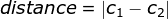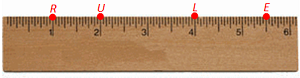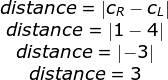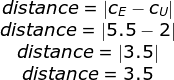# Ruler Postulate: Definition & Examples

Lesson Transcript
Instructor: Miriam Snare

Miriam has taught middle- and high-school math for over 10 years and has a master's degree in Curriculum and Instruction.

This lesson explains the definition of the ruler postulate. You'll see several examples that use the ruler postulate. Then, you can check your knowledge with a quiz.

## Definition of the Ruler Postulate

The ruler postulate tells us how to find the distance between two points. First, we have to match each of the two points to a number. We call these numbers the coordinates of the points. Then, we subtract the coordinates from each other. If the resulting difference is negative, we take the absolute value of the result because the distance is always a positive number. We can see this process in the following formula:Distance is equal to the absolute value of C1 and C2 where C1 and C2 stand for the coordinates of the two points. It does not matter in which order you put the coordinates into the formula.

An error occurred trying to load this video.

Try refreshing the page, or contact customer support.

Coming up next: What is a Fact Family? - Definition & Examples

### You're on a roll. Keep up the good work!

Replay
Your next lesson will play in 10 seconds
• 0:00 Definition of the…
• 0:44 The Ruler Postulate on a Ruler
• 3:04 The Ruler Postulate on…
• 4:45 Lesson Summary
Save Save

Want to watch this again later?

Log in or sign up to add this lesson to a Custom Course.

Timeline
Autoplay
Autoplay
Speed Speed

## The Ruler Postulate on a Ruler

Believe it or not, the ruler postulate actually has to do with using a ruler. The ruler postulate doesn't care whether you're using a ruler with inches or centimeters (or some other measuring system you want to create). When you use the ruler postulate on a ruler, you are not using the ruler in the normal way that you usually measure between two points. Usually, we line up the zero with one point and then see where the other point falls on the ruler. With the ruler postulate, we are just using the ruler to give each point a coordinate. So, we line up the ruler along all of the points at one time and assign each point the number on the ruler that it falls on. Just make sure the ruler is long enough to include all of the points that you are working with so that each point ends up with a coordinate.

Let's start with a couple of examples with points on a ruler. Here, we have a diagram of a ruler with points R, U, L, and E. Each point is matched with a coordinate: R is matched with 1, U is matched with 2, L is matched to 4, and E is matched to 5.5.Now, let's find the distance between point R and point L. We will fill in the ruler postulate formula with the coordinates for R and L and simplify:So, the distance from R to L is 3 units. Notice that I didn't say inches or millimeters. We're not using the ruler to measure length. Distance here is about the number of units between coordinates - no matter what those units are.

Let's find the distance between another pair of points on the ruler: point E and point U. We will fill in the ruler postulate formula with the coordinates for E and U and simplify:So, the distance from E to U is 3.5 units.

To unlock this lesson you must be a Study.com Member.
Create your account

### Register to view this lesson

Are you a student or a teacher?

### Unlock Your Education

#### See for yourself why 30 million people use Study.com

##### Become a Study.com member and start learning now.
Back
What teachers are saying about Study.com
Create an account to start this course today
Used by over 30 million students worldwide